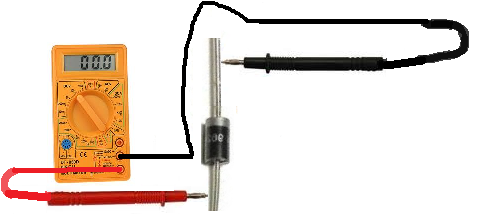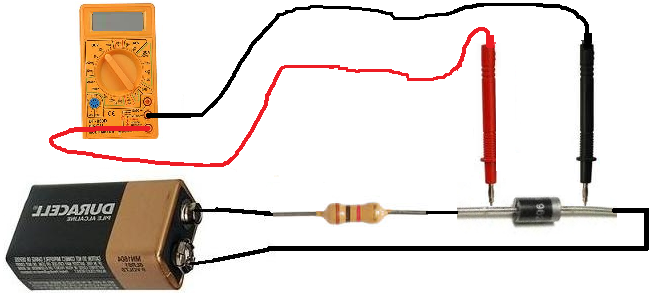﻿ How to Test a Diode# How To Test a DiodeIn this article, we will go over different tests that we can use to check whether a diode is good or not, all by utilizing the functions of a digital multimeter.

There are many checks we can do to see if a diode is functioning the way it should. We will use and exploit the characteristics and behaviors that a diode should show if it is good and, in thus doing so, determine whether is good or defective.

So let's start:

### How to Test a Diode with the Ohmmeter of a Multimeter

A very good test you can do is to check a diode with your multimeter set to the ohmmeter setting.

This is a simple test we can do to check whether it is good, open, or shorted.

So we take the ohmmeter and place it across the leads of the diode. The orientation is very important.

### Anode-Cathode Diode Resistance Test

We first take the ohmmeter and place the positive probe on the anode of the diode (the black part of the diode_ and the negative probe on the cathode of the diode (the silver strip), as shown above. In this setup, the diode should read a moderately low resistance, maybe a few tens of thousands or low hundreds of thousands of ohms. For example, you may read 230KΩ.### Cathode-Anode Diode Resistance Test

Now take the ohmmeter and switch the probes around so that the positive probe of the multimeter is now on the cathode of the diode and the negative lead on the anode. In this setup now, the diode should read a much higher resistance, over 1MΩ. It may even indicate 'OL' for an open circuit, since the resistance is so high.If you read a moderately low resistance with the leads on the diode one way and a high resistance with the leads the other, this is a sign that the diode is good. A diode should read relatively low resistance in the forward biased direction and very high resistance in the reverse biased direction.

### Open Diode

If the diode reads high resistance in both directions, this is a sign that the diode is open. A diode should not measure very high resistance in the forward biased direction. The diode should be replaced in the circuit.

### Shorted Diode

If the diode reads low resistances in both directions, this is a sign that the diode is shorted. A diode should not measure low resistance in the reverse biased direction. The diode should be replaced in the circuit.

### How to Test a Diode with the Voltmeter of a Multimeter

A second test you can do to check is a diode is with a voltmeter of a multimeter (or simply just a voltmeter if you have one.)

Because diodes drop a specific voltage across their terminals with their threshold voltage is exceeded, we can use these properties to see if a diode is reading a healthy and correct voltage across their terminals.

To conduct this test, we need to place the diode in a circuit with DC voltage feeding the diode.

The circuit below is a good test to check a diode:You can feed the diode any voltage you want above the threshold voltage. And the diode will drop the threshold voltage across its terminals.

The diode you are most likely using is a silicon diode. Silicon diodes drop approximately 0.6V-0.7v across their terminals. So when measuring voltage across the leads of the diodes, you should read a voltage in this range. If you do, the diode is reading a healthy voltage and should be good. If the diode is a germanium diode, then the voltage dropped across it should be anywhere in the proximity of 0.3V.

Make sure that you use a resistor when testing the diode with voltage, as to not make the diode overheat. Do not hook up the voltage directly to the diode. Use a resistor in between. Any resistor value such as 1KΩ or in that range will suffice.

### Open Diode

If you reading a very high voltage across the diode, such as the voltage you are supplying it, the diode is open and, thus, defective, and should be replaced.

### Shorted Diode

If you reading no to very little voltage across the diode, then the diode is shorted and should be replaced.

So there you have it, these are 2 strong tests you can do to test whether a diode is good or not.

Related Resources

How to Test a Zener Diode